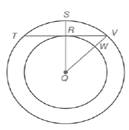Chapter 6.1, Problem 17EElementary Geometry For College St...

7th Edition
Alexander + 2 others
ISBN: 9781337614085

Solutions

Chapter
SectionElementary Geometry For College St...

7th Edition
Alexander + 2 others
ISBN: 9781337614085
Textbook Problem

Given: Concentric circles with center Q T V = 8  and  V W = 2 R Q ¯ ⊥ T V ¯Exercises 16, 17 Find: R Q (HINT: Let R Q = x . )

To determine

To calculate:

The value of RQ by using the provided figure.

Explanation

Given:

The concentric circles with centre Q, RQ¯TV¯, TV=8 and VW=2

Postulate used:

A radius that is perpendicular to a chord bisects the chord.

Concentric circle:

Concentric circle are coplanar circles that have a common centre.

Since, a radius is a line segment that joins the centre of the circle to a point on a circle.

Calculation:

Let, RQ=x

Since, RQ¯TV¯ then RT=TV where TV=8 and VW=2.

Now, RV=12TV then we obtain

Still sussing out bartleby?

Check out a sample textbook solution.

See a sample solution

The Solution to Your Study Problems

Bartleby provides explanations to thousands of textbook problems written by our experts, many with advanced degrees!

Get Started

In Exercises 4756, solve the given equation for the indicated variable. 423x=256

Finite Mathematics and Applied Calculus (MindTap Course List)

In Problems 5-10, find the slope of the line passing through the given pair of points.

Mathematical Applications for the Management, Life, and Social Sciences

Evaluate limx01+tanx1+sinxx3.

Single Variable Calculus: Early Transcendentals

n=12(14)n converges to: a) 94 b) 2 c) 23 d) the series diverges

Study Guide for Stewart's Single Variable Calculus: Early Transcendentals, 8th

True or False:

Study Guide for Stewart's Multivariable Calculus, 8th